This is a method of adding two numbers using small progressive steps along a line.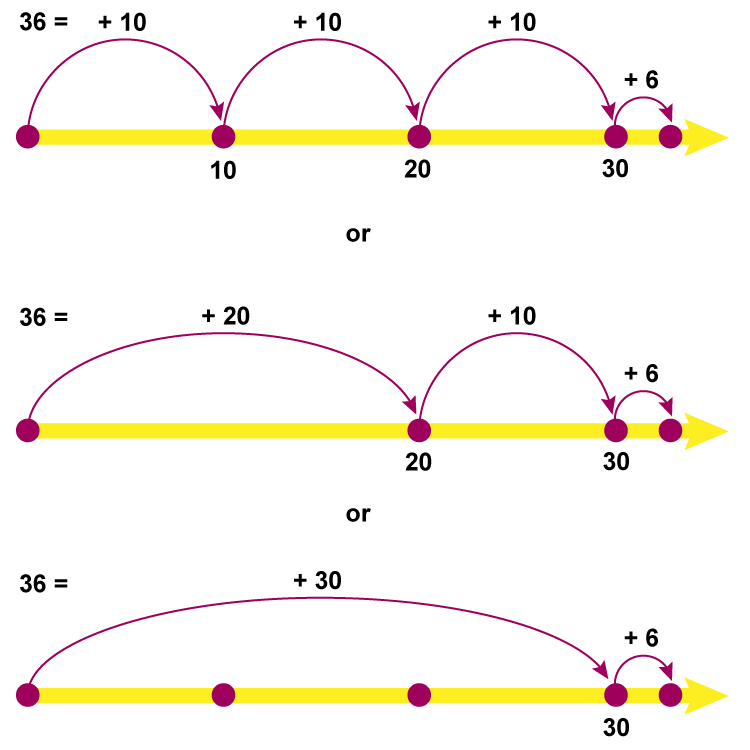Example 1

42+27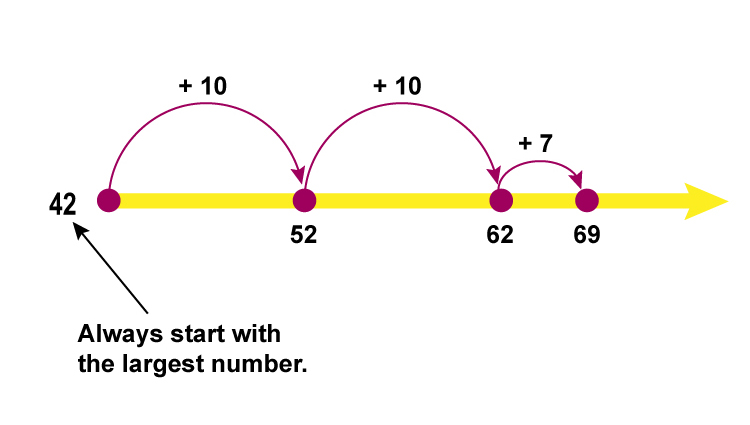To see how this is broken down

## Step by step

Example 1

42+27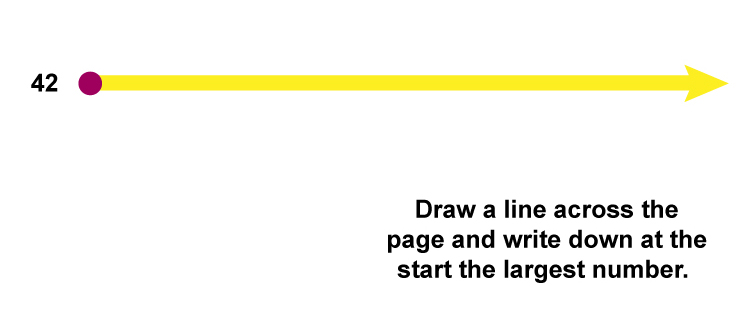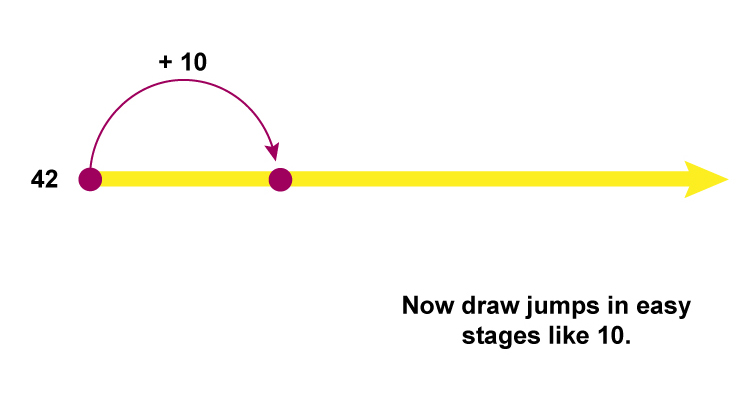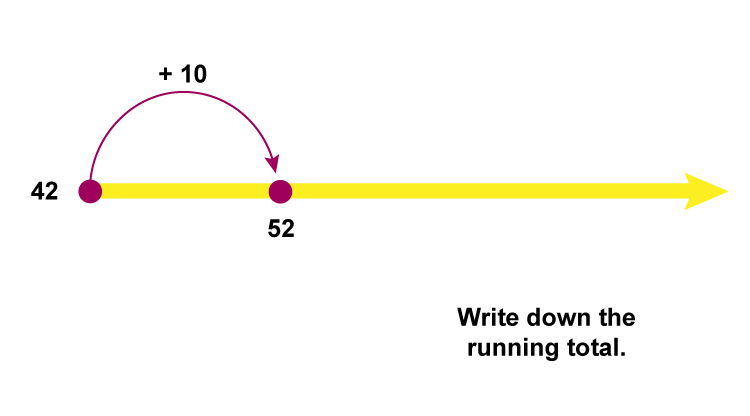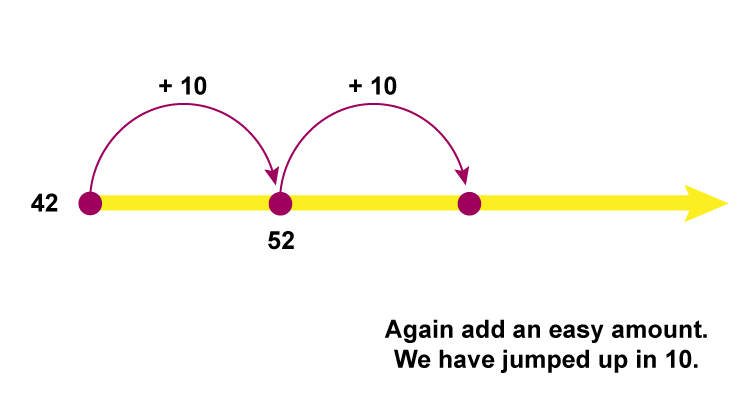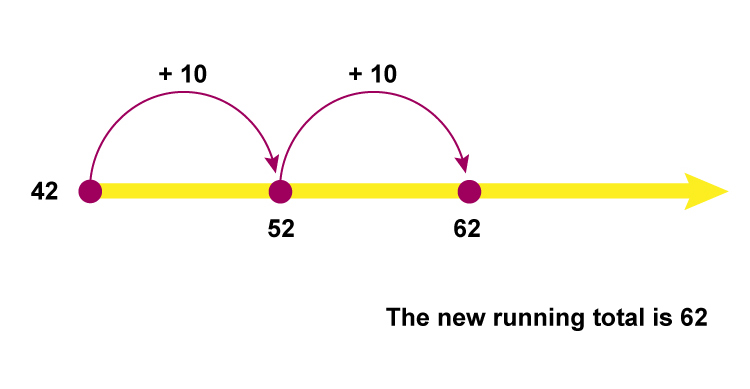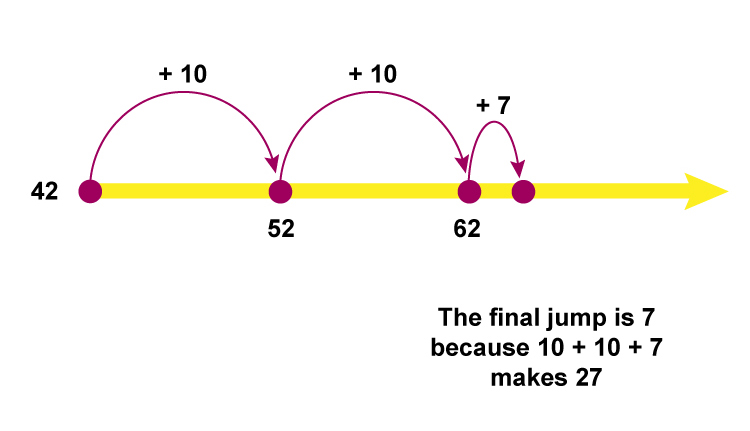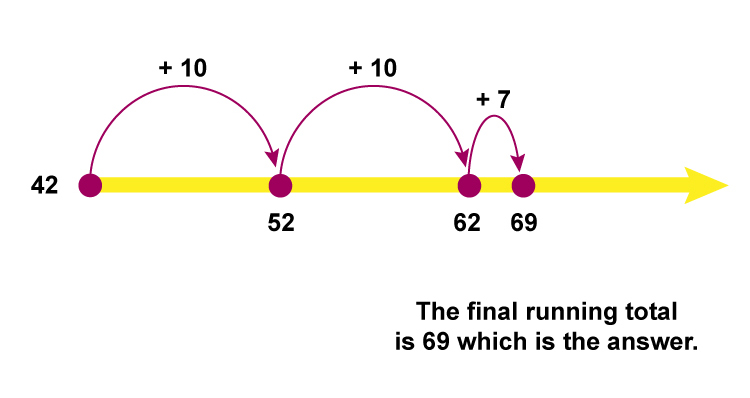Answer: =69

Answer: =69

Example 2

419+275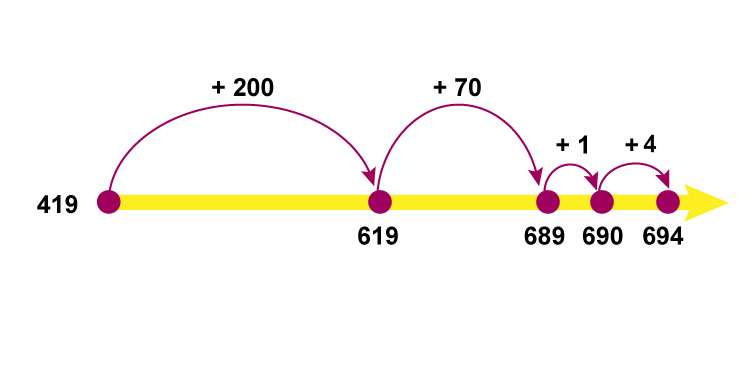To see how this is broken down

## Step by step

Example 2

419+275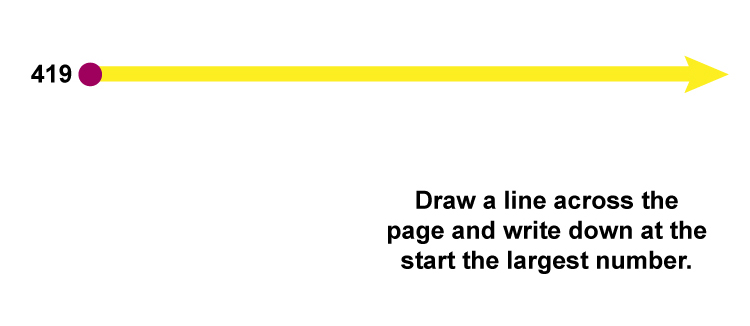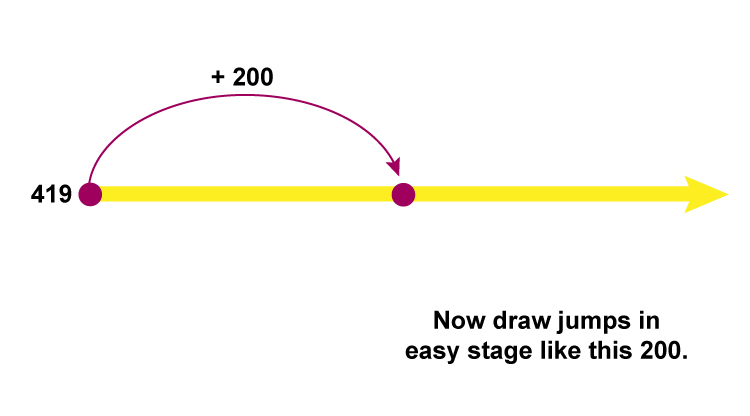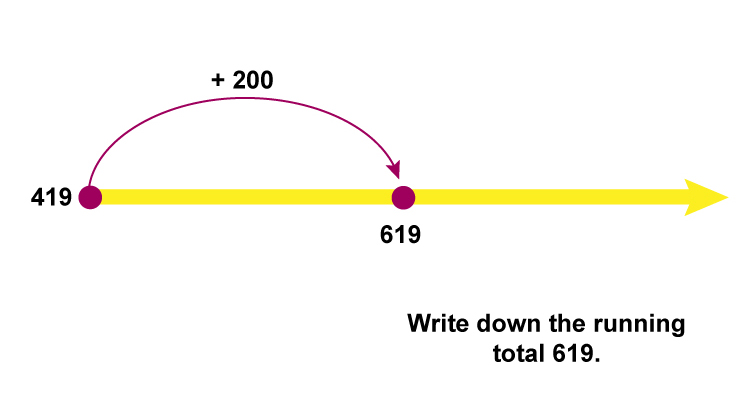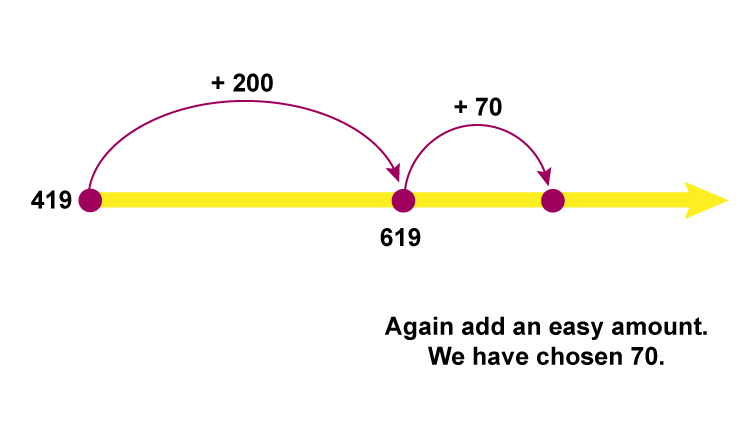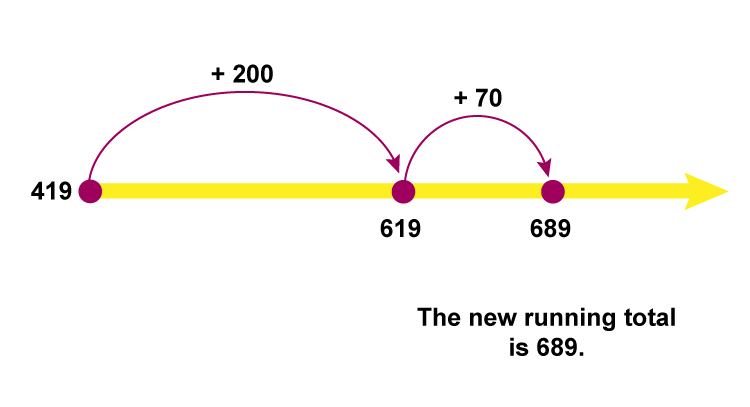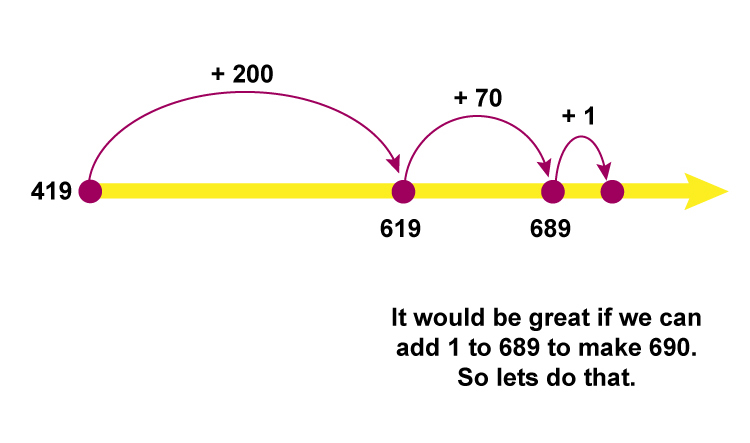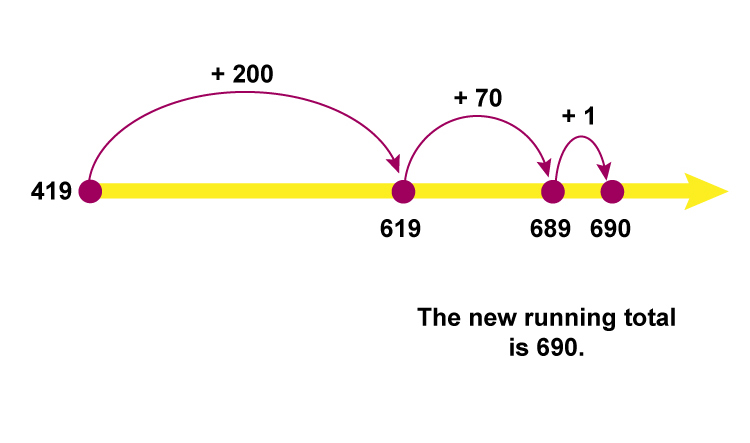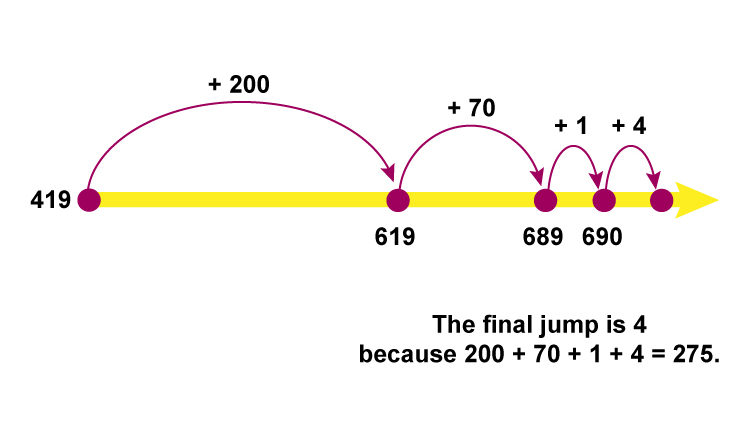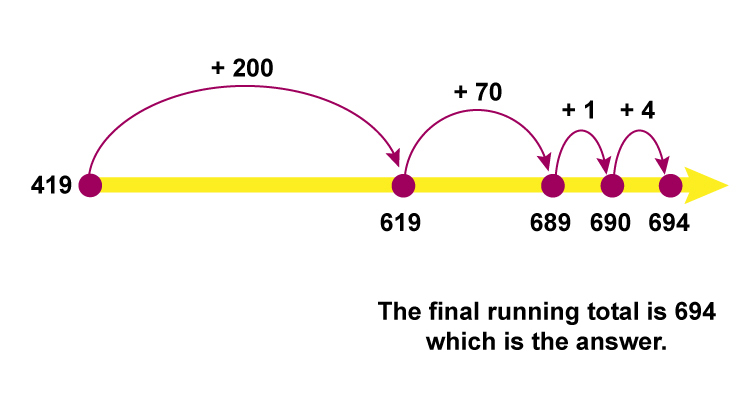Answer: =964

Example 3

256+137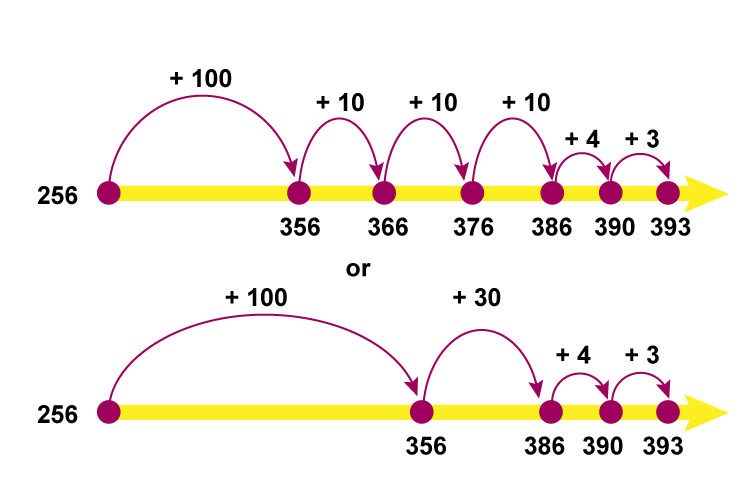To see how this is broken down

## Step by step

Example 3

256+137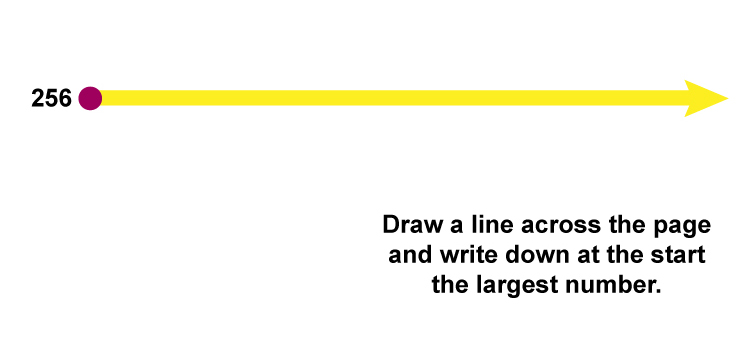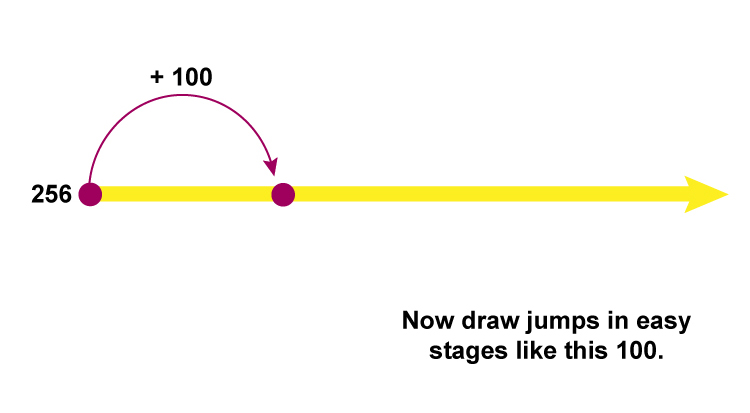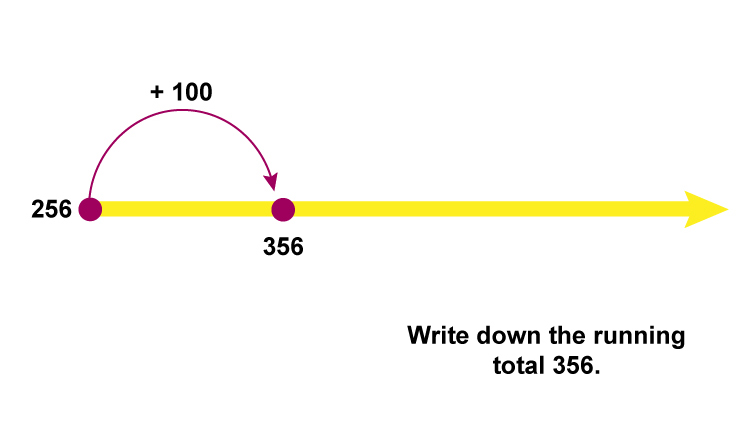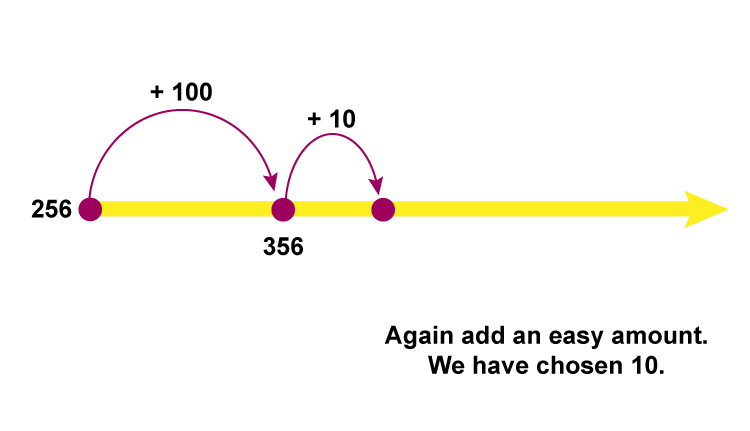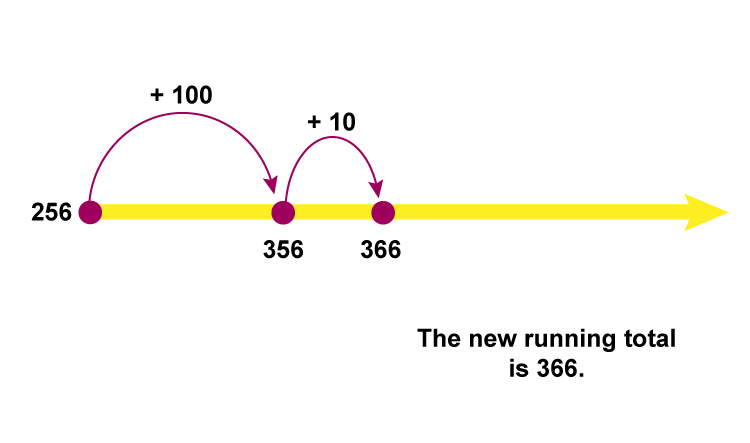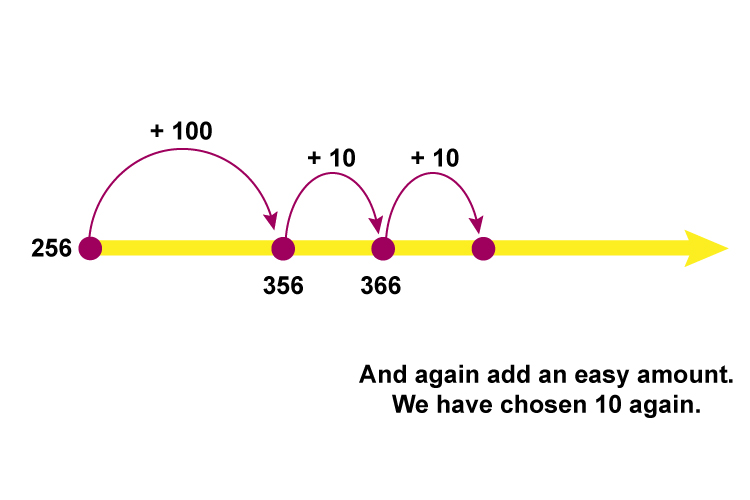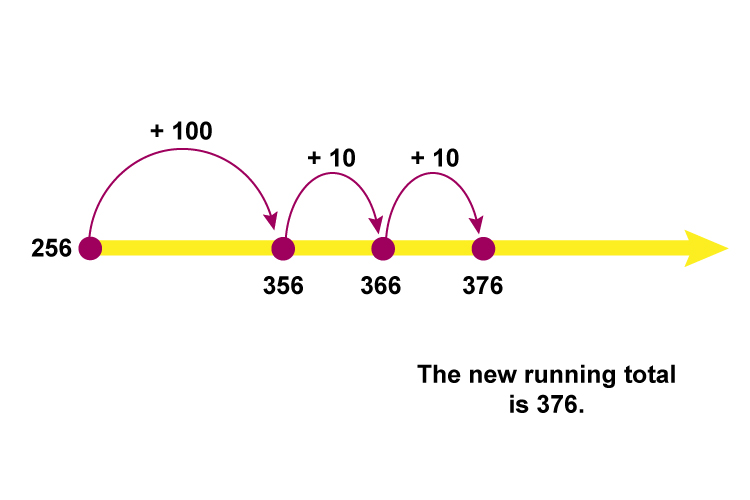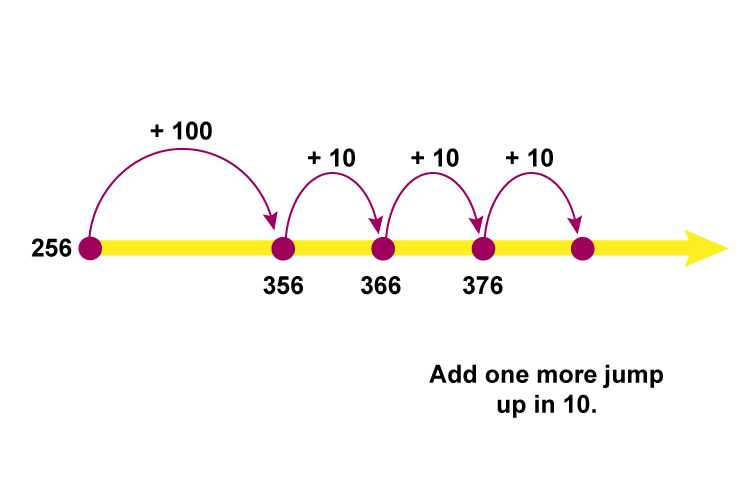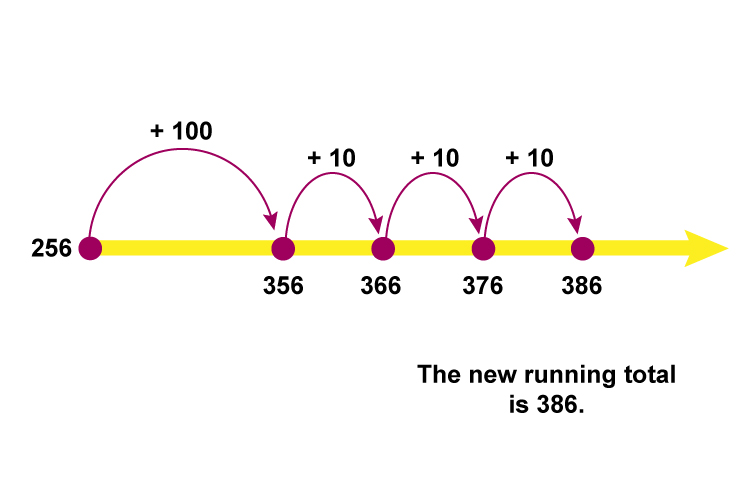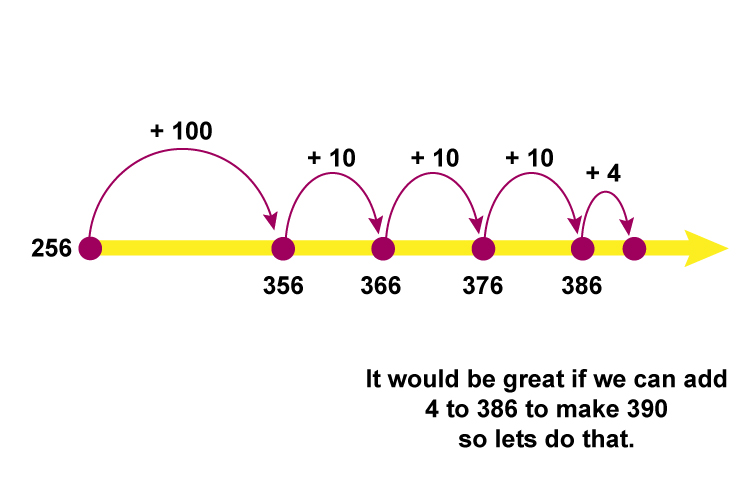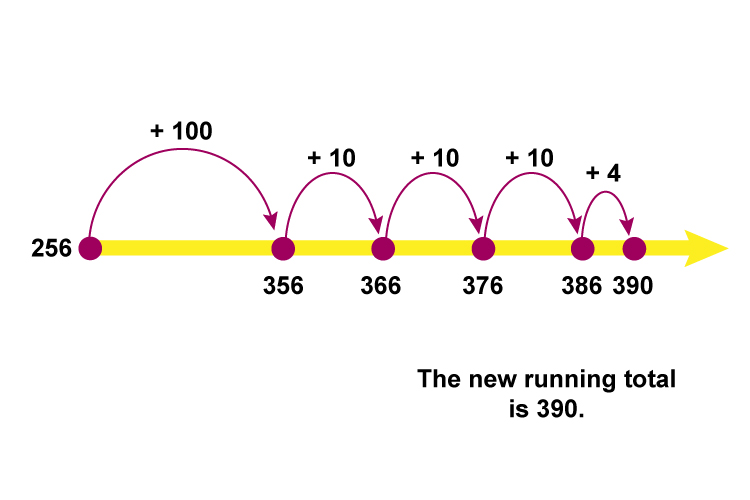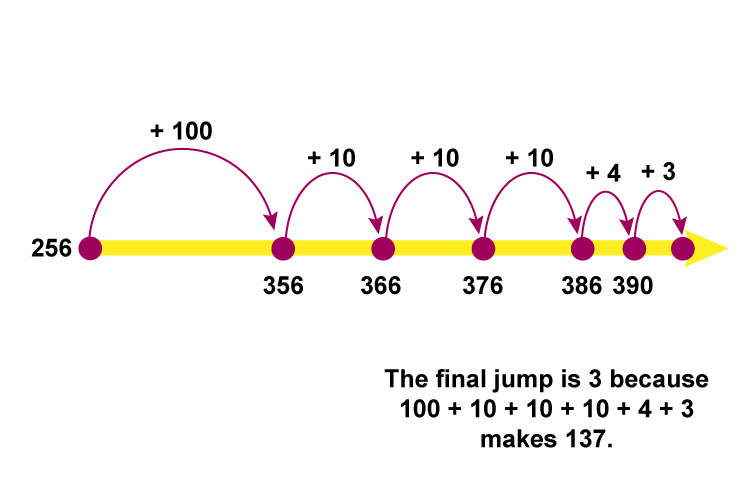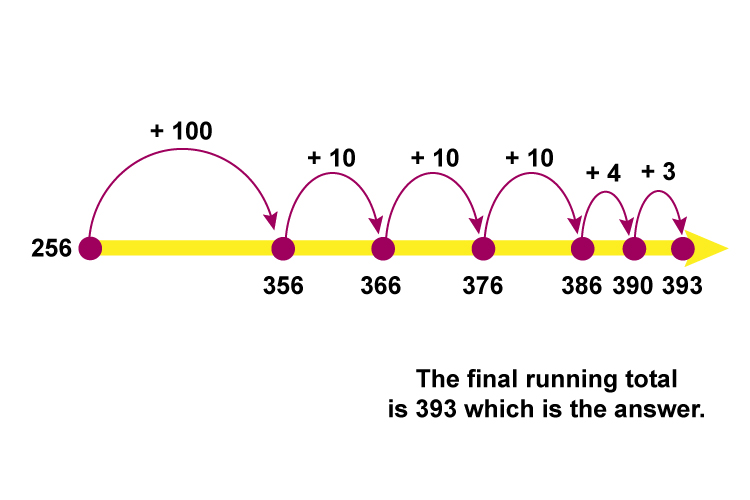Answer: 256+137=393

Answer: =393